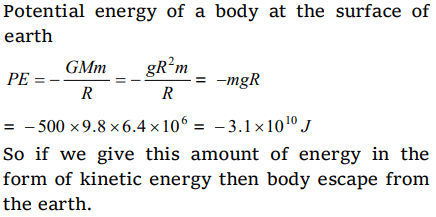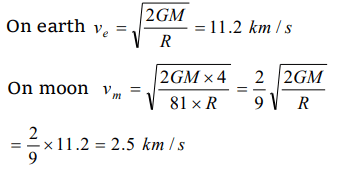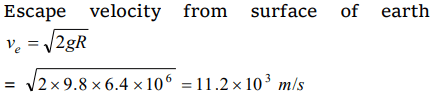## Gravitation Questions and Answers Part-15

1. The escape velocity from the surface of earth is $V_{e}$ . The escape velocity from the surface of a planet whose mass and radius are 3 times those of the earth will be
a) $V_{e}$
b) $3 V_{e}$
c) $9 V_{e}$
d) $27 V_{e}$

Explanation:2.How much energy will be necessary for making a body of 500 kg escape from the earth [g = 9.8 m/s2, radius of earth = 6.4 * 106 m]
a) About $9.8\times 10^{6}J$
b) About $6.4\times 10^{8}J$
c) About $3.1\times 10^{10}J$
d) About $27.4\times 10^{12}J$

Explanation:3. The escape velocity for the earth is 11.2 km/sec. The mass of another planet is 100 times that of the earth and its radius is 4 times that of the earth. The escape velocity for this planet will be
a) 112.0 km/s
b) 5.6 km/s
c) 280.0 km/s
d) 56.0 km/s

Explanation:4. The escape velocity of a planet having mass 6 times and radius 2 times as that of earth is
a) $\sqrt{3} V_{e}$
b) $3 V_{e}$
c) $\sqrt{2} V_{e}$
d) $2 V_{e}$

Explanation:5. The escape velocity of an object on a planet whose g value is 9 times on earth and whose radius is 4 times that of earth in km/s is
a) 67.2
b) 33.6
c) 16.8
d) 25.2

Explanation:6. The escape velocity on earth is 11.2 km/s. On another planet having twice radius and 8 times mass of the earth, the escape velocity will be
a) 3.7 km/s
b) 11.2 km/s
c) 22.4 km/s
d) 42.4 km/s

Explanation:7.The escape velocity of a body on the surface of the earth is 11.2 km/s. If the earth's mass increases to twice its present value and the radius of the earth becomes half, the escape velocity would become
a) 5.6 km/s
b) 11.2 km/s (remain unchanged)
c) 22.4 km/s
d) 44.8 km/s

Explanation:8. Given mass of the moon is 1/81 of the mass of the earth and corresponding radius is 1/4 of the earth. If escape velocity on the earth surface is 11.2 km/s, the value of same on the surface of the moon is
a) 0.14 km/s
b) 0.5 km/s
c) 2.5 km/s
d) 5 km/s

Explanation:9. The angular velocity of rotation of star (of mass M and radius R) at which the matter start to escape from its equator will be
a) $\sqrt{\frac{2GM^{2}}{R}}$
b) $\sqrt{\frac{2GM}{g}}$
c) $\sqrt{\frac{2GM}{R^{3}}}$
d) $\sqrt{\frac{2GR}{M}}$10. The least velocity required to throw a body away from the surface of a planet so that it may not return is (radius of the planet is $6.4\times10^{6} m,g=9.8 m/sec^{2}$     )
a) $9.8\times10^{-3} m/sec$
b) $12.8\times10^{3} m/sec$
c) $9.8\times10^{3} m/sec$
d) $11.2\times10^{3} m/sec$